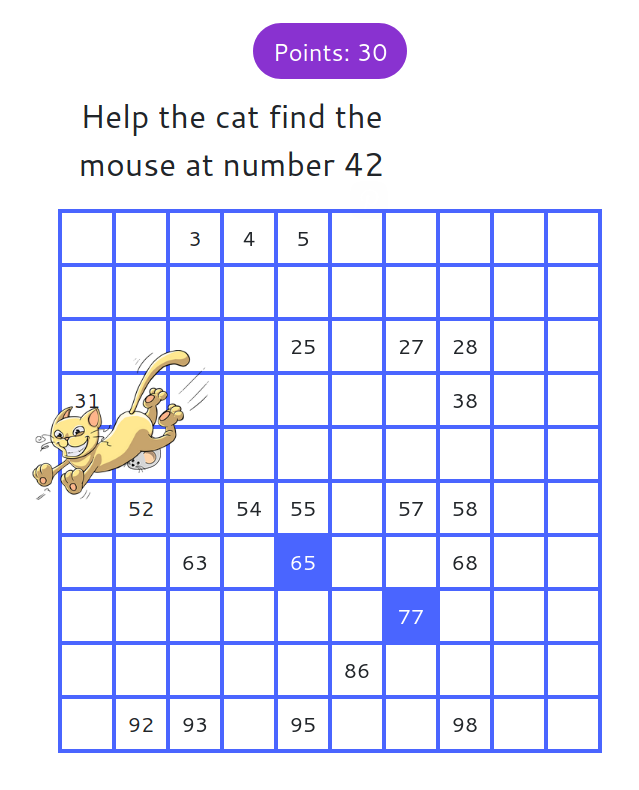MM Practice
×
Multiplication
Division
Place Value
Fractions & Decimals
Measurement
Statistics & Probability
Pre-Algebra
Money
Kindergarten
Geometry

# Give the Cat a Mouse: a 100-Chart GameOnline practice for grades K-3

In this game, you are asked to find a specific number on a 100-chart. This helps students understand two-digit numbers and how numbers are arranged in a 100-chart by tens and ones.

The options allow you to change the starting numbers and the step, allowing you to create, not only a chart from 1-100, but others such as a chart from 0 to 990 by tens, or a chart from 1 to 199 by twos, etc. Trying other options makes the game more challenging. :)

You can also choose how many numbers you want to find and the percentage of numbers that are already pre-filled in the chart.

In "mystery mode", the computer chooses a random starting number (between 1 and 100) and a random step (between 1 and 10), and the chart is initially totally empty. Enjoy the challenge!

Screenshot:Points: 0Allow my comment to be posted on this site